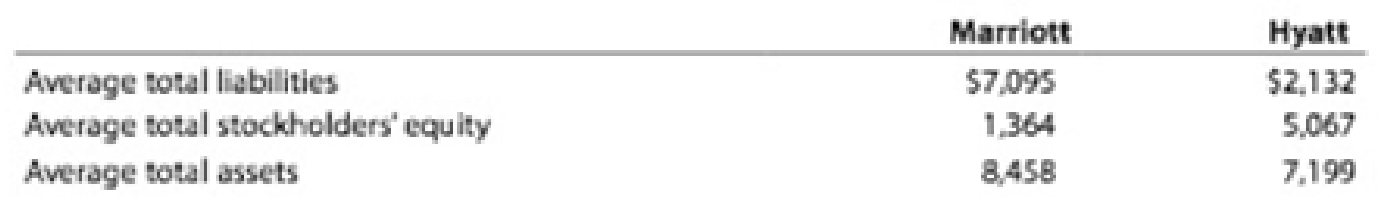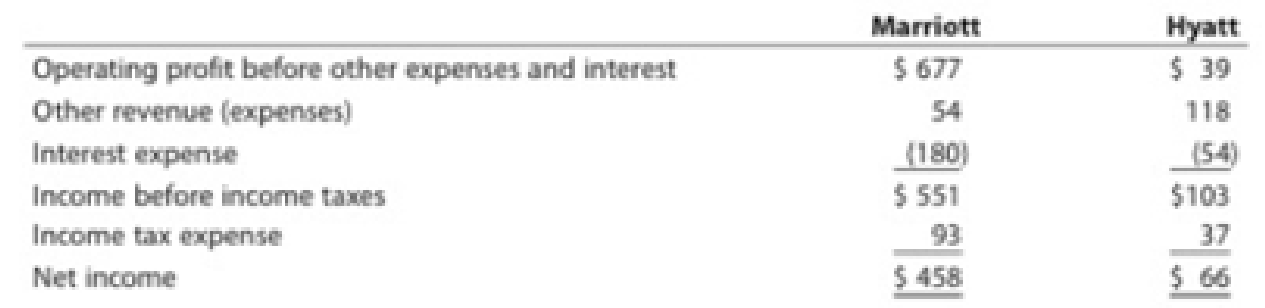Chapter 17, Problem 6CP

Chapter
Section
Textbook Problem
1 views

# The average liabilities, average stockholders' equity, and average total assets are as follows:1. Determine the following ratios for both companies, rounding ratios and percentages to one decimal place: a. Return on total assets b. Return on stockholders' equity c. Times interest earned d. Ratio of total liabilities to stockholders' equity 2. Based on the information in (1), analyze and compare the two companies' solvency and profitability.Comprehensive profitability and solvency analysisMarriott International, Inc., and Hyatt Hotels Corporation are two major owners and managers of lodging and resort properties in the United States. Abstracted income statement information for the two companies is as follows for a recent year (in millions):Balance sheet information is as follows:1.

To determine

Determine the following ratios for three years:

1. (a) Return on total assets
2. (b) Return on stockholders’ equity
3. (c) Times interest earned ratio
4. (d) Ratio of total liabilities to stockholders’ equity
Explanation

Financial Ratios: Financial ratios are the metrics used to evaluate the liquidity, capabilities, profitability, and overall performance of a company.

a)

Return on total assets for M and H.

Rate of return on assets (Company M)=Netincome + Interest expenseAverage total assets=$458+$180$8,458=7.5% Rate of return on assets (Company H)=Netincome + Interest expenseAverage total assets=$66+$54$7199=1.7%

Description:

Return on assets determines the particular company’s overall earning power. It is determined by dividing sum of net income and interest expense and average total assets.

Formula:

Rate of return on assets=Netincome + Interest expenseAverage total assets

Hence, rate of return on assets for M and H are 7.5% and 1.7% respectively.

b)

Return on stockholders’ equity for M and H.

Rate of return on stockholders' equity(Company M)}= Net income Average stockholder’s equity=$458$1,364=33.6%

Rate of return on stockholders' equity(Company H)}= Net income Average stockholder’s equity=$66$5,067=1.3%

Description:

Rate of return on stockholders’ equity is used to determine the relationship between the net income and the average common equity that are invested in the company.

Formula: Rate of return on stockholders' equtiy = Net incomeAverage  stockholder’s equity

Hence, rate of return on stockholders’ equity for Company M and Company H are 33.6% and 1.3% respectively.

c)

Times interest earned ratio for Company M and Company H

Times-interest-earned ratio(Company M) }=Income before income tax+Interest expenseInterest expense=$551+$180\$180=4

2.

To determine

Analyze and compare two company’s solvency position and profitability position

### Still sussing out bartleby?

Check out a sample textbook solution.

See a sample solution

#### The Solution to Your Study Problems

Bartleby provides explanations to thousands of textbook problems written by our experts, many with advanced degrees!

Get Started

#### Why is productivity important?

Brief Principles of Macroeconomics (MindTap Course List)

#### Give an example of each form.

Foundations of Business (MindTap Course List)

#### List and describe six costs of inflation.

Principles of Economics (MindTap Course List)

#### What is inflation and what causes it?

Essentials of Economics (MindTap Course List)

#### What is meant by controlling?

Managerial Accounting: The Cornerstone of Business Decision-Making

#### WORKING CAPITAL INVESTMENT Prestopino Corporation produces motorcycle batteries. Prestopino turns out 1,500 bat...

Fundamentals of Financial Management, Concise Edition (with Thomson ONE - Business School Edition, 1 term (6 months) Printed Access Card) (MindTap Course List)